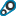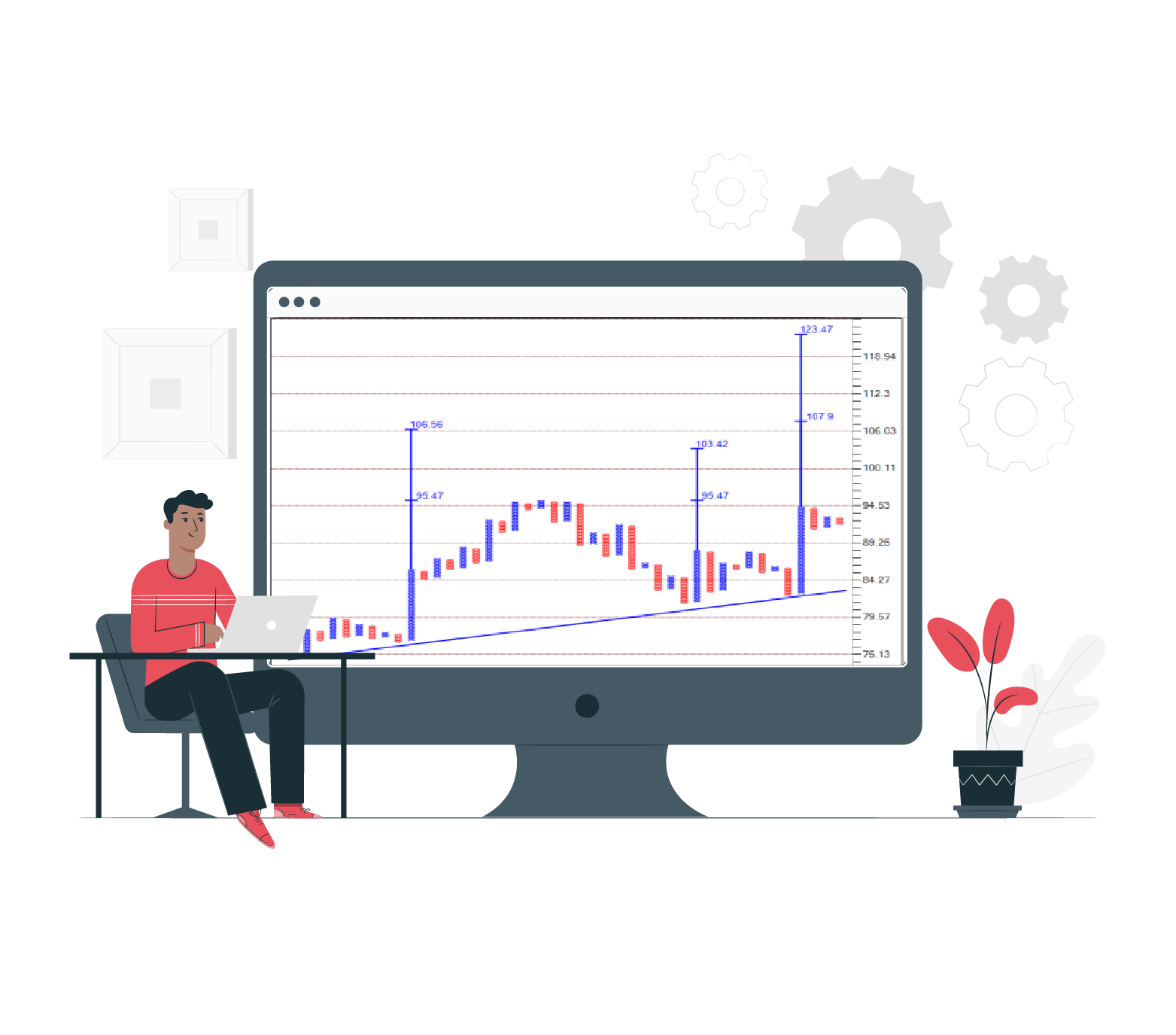17 CoursesPoint & Figure charts
Preview CoursePoint & Figure charts
Preview CoursePoint & Figure charts
Preview Course

Point & Figure charts

##### Out of Box: A very effective stock selection method.

Out of Box: Pattern Matrix and Counter. A very effective & innovative method of Stock selection.Point & Figure charts
Preview Course

Point & Figure charts

##### Learn basics of Point & Figure charts

Learn basics of Point & Figure charts. Construction and basic patterns.Point & Figure charts
Preview Course

Point & Figure charts

##### P&F Construction Exercise

Learn how P&F charts are drawn. Step wise explained in excel.Point & Figure charts
Preview Course

Point & Figure charts

A strategy to trade considering price setup on multiple timeframesPoint & Figure charts
Preview Course

Point & Figure charts

##### T20 Setup explained

T20 Setup explainedPoint & Figure charts
Preview Course

Point & Figure chartsPoint & Figure charts
Preview Course

Point & Figure charts

##### P&F Setup

A simple and logical way to know state of the market.Point & Figure charts
Preview Course

Point & Figure charts

##### Horizontal Counts

How to plot Horizontal counts in P&F chartsPoint & Figure charts
Preview Course

Point & Figure charts

##### How to trade Options using P&F Multi-column breakout method

How to trade Options using P&F Multi-column breakout method. Objective entry and exit method to buy Options.Point & Figure charts
Preview Course

Point & Figure charts

##### Multi-time frame analysis & Level Picker

Multi-time frame analysis and Level PickerPoint & Figure charts
Preview Course

Point & Figure charts

##### Anchor Column Strike

Know what is Anchor column strike patternPoint & Figure charts
Preview Course

Point & Figure charts

##### Anchor Lines

How to trade Anchor Support & Resistance linesPoint & Figure charts
Preview Course

Point & Figure charts

What is Pair Trading and How to do itPoint & Figure charts
Preview Course

Point & Figure charts

##### Webinar: Chart Queries & discussion

Webinar conducted by Prashant Shah to discuss chart queries, Price, Relative Strength and Breadth analysisPoint & Figure charts
Preview Course

Point & Figure charts